# Schlumberger

## Analyst Listing

The following analysts provide coverage for the subject firm as of May 2016:

 Broker Analyst Analyst Email Atlantic Equities Barry MacCarthy b.maccarthy@atlantic-equities.com Piper Jaffray Bill Herbert william.a.herbert@simmonspjc.com Scotia Howard Weil Bill Sanchez william.sanchez@scotiabank.com Jefferies Brad Handler brad.handler@jefferies.com William Blair Brandon Dobell bdobell@williamblair.com GMP Securities Brian Uhlmer buhlmer@gmpsecurities.com Tudor Pickering Holt & Co. Byron K. Pope bpope@tudorpickering.com Susquehanna Financial Group Charles Minervino charles.minervino@sig.com BMO Capital Markets Daniel Boyd daniel.boyd@bmo.com Johnson Rice & Company Daniel Burke dburke@jrco.com KLR Group Darren Gacicia dfg@klrgroup.com Societe Generale Edward Muztafago edward.muztafago@sgcib.com Raymond James J .Marshall Adkins marshall.adkins@raymondjames.com Evercore ISI James West james.west@evercoreisi.com Credit Suisse James Wicklund james.wicklund@credit-suisse.com Wells Fargo Securities Judson E. Bailey jud.bailey@wellsfargo.com Seaport Global Securities Ken Sill ksill@seaportglobal.com Griffin Securities Kevin Simpson ksimpson@griffinsecurities.com RBC Capital Markets Kurt Hallead kurt.hallead@rbccm.com Capital One Securities Luke M. Lemoine luke.lemoine@capitalone.com Cowen & Company Marc Bianchi marc.bianchi@cowen.com Nomura Research Matthew Johnston matthew.johnston@nomura.com Stephens Inc Matthew Marietta matt.marietta@stephens.com Guggenheim Securities Michael LaMotte michael.lamotte@guggenheimpartners.com Clarksons Platou Securities Nokta Haithum haithum.nokta@clarksons.com IBERIA Capital Partners Robert Mackenzie robert.mackenzie@iberiabank.com KeyBanc Capital Markets Robin Shoemaker robin_shoemaker@key.com DA Davidson Sonny Randhawa srandhawa@dadco.com FBR Capital Markets & Co Thomas Curran tcurran@fbr.com

## Primary Input Data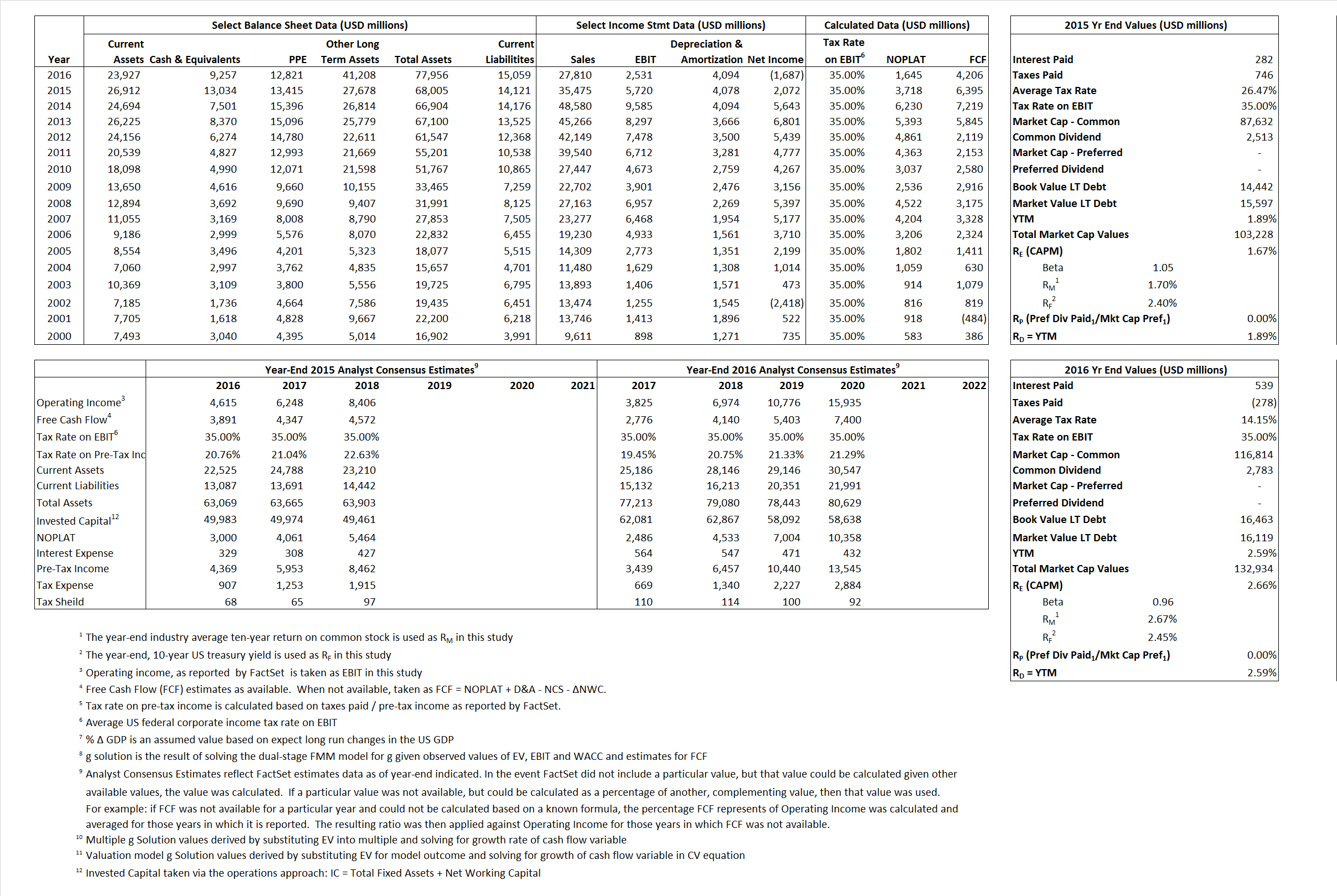## Derived Input Data

### Equational Form

Net Operating Profit Less Adjusted Taxes NOPLAT   3,718  1,645$NOPLAT\, =\, EBIT\, x\, (1 \,-\, Avg \,\,Tax\,\, Rate\,\, on\,\, EBIT)$
Free Cash Flow FCF  6,395  4,206$FCF\,=NOPLAT\,+\,Non-Cash\,Expenses-\Delta NWC\,-\,NCS$
Tax Shield TS  75  76$TS\,=\,Interest\,\,Paid\,\,x\,\, Avg \,\,Tax\,\,Rate\,\, on\,\, Pre-Tax\,\, Income$
Invested Capital IC  53,884  62,897$IC\,=\,Fixed\,\,Operating\,\,Assets\,\,+\,\,Net\,\, Working\,\, Capital$
Return on Invested Capital ROIC 6.90% 2.62%$ROIC\,=\,\frac { NOPLAT }{ IC }$
Net Investment NetInv  5,234  13,107$NetInv\,=\,{ {IC}_{1}}-{{IC}_{0}}+Depreciation$
Investment Rate IR 140.77% 796.71%$IR\,=\,\frac {NetInv}{NOPLAT}$
Weighted Average Cost of Capital WACCMarket 1.62% 2.61%$WACC\,=\,\frac { E }{ V } { R }_{ E }\,+\,\frac { P }{ V } { R }_{ P }\,+\,\frac { D }{ V } { R }_{ D }\left( 1- Avg\,\, Tax\,\,Rate\,\,on\,\,Pre-Tax\,\,Income \right)$
WACCBook  8.33% 7.82%
Enterprise value EVMarket  90,194  123,677$EV\,=\,Market\,\,Cap\,\,Equity\,+\,\,Long\,\,Term\,\,Debt\,-\,Cash$
EVBook  92,817  124,020
EV/EBIT Multiple$\frac{EV_{Market}}{EBIT}$  15.77  48.86$EV/EBIT\,=\,\frac { EV}{ EBIT}$
Long-Run Growth g = IR x ROIC
9.71%   20.84% Long-run growth rates of the income variable  are used in the Continuing Value portion of the valuation models.
g = %$\Delta$ GDP   2.50%   2.50%

## Valuation Model Outcomes

The outcomes presented in this study are the result of original input data, derived data, and synthesized inputs and, depending on the equational form of any particular valuation model, may result in irrelevant or implausible results.  For example, in the event WACC < g, the value of this term, often found in the denominator of an equation’s continuation value term, will be expressly negative and may result in a negative overall valuation for the firm.  In the event of a WACC < g relation, the model form as applied to the subject firm offers an irrelevant outcome.

### Equational form

Key Value Driver (NOPLAT) KVD (NOPLAT)${ Value }_{ DCF/KVD }=\sum { \frac { NOPLAT_{ t } }{ { \left( 1+WACC \right) }^{ t } } +\frac { \frac { { NOPLAT }_{ 1 }\left( 1-\frac { g }{ ROIC } \right) }{ WACC-g } }{ { \left( 1+WACC \right) }^{ t } } }$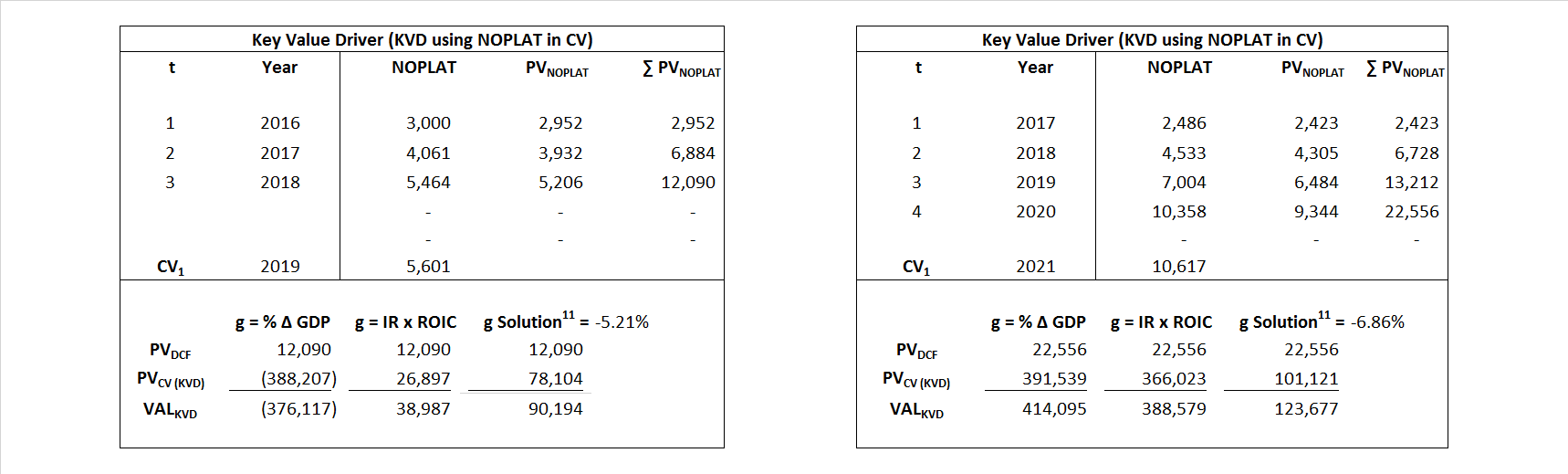Key Value Driver (FCF) KVD (FCF)${ Value }_{ DCF/KVD }=\sum { \frac { FCF_{ t } }{ { \left( 1+WACC \right) }^{ t } } +\frac { \frac { { NOPLAT }_{ 1 }\left( 1-\frac { g }{ ROIC } \right) }{ WACC-g } }{ { \left( 1+WACC \right) }^{ t } } }$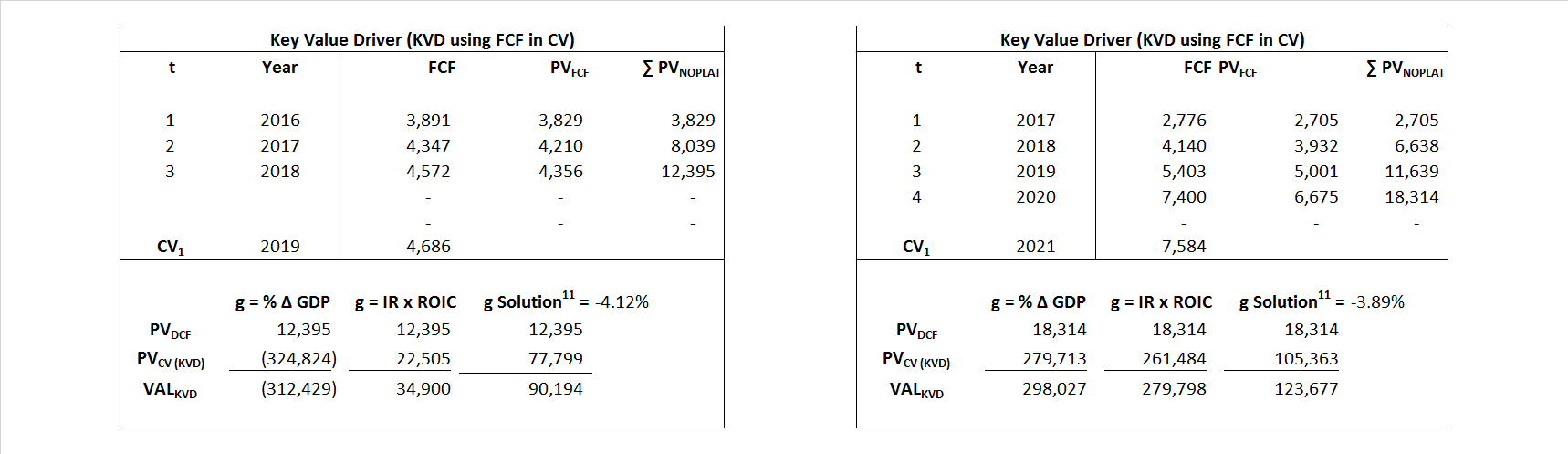Free Cash Flow FCF${ Value }_{ DCF/FCF }=\sum { \frac { FCF_{ t } }{ { \left( 1+WACC \right) }^{ t } } +\frac { \frac { { FCF }_{ 1 }}{ WACC-g } }{ { \left( 1+WACC \right) }^{ t } } }$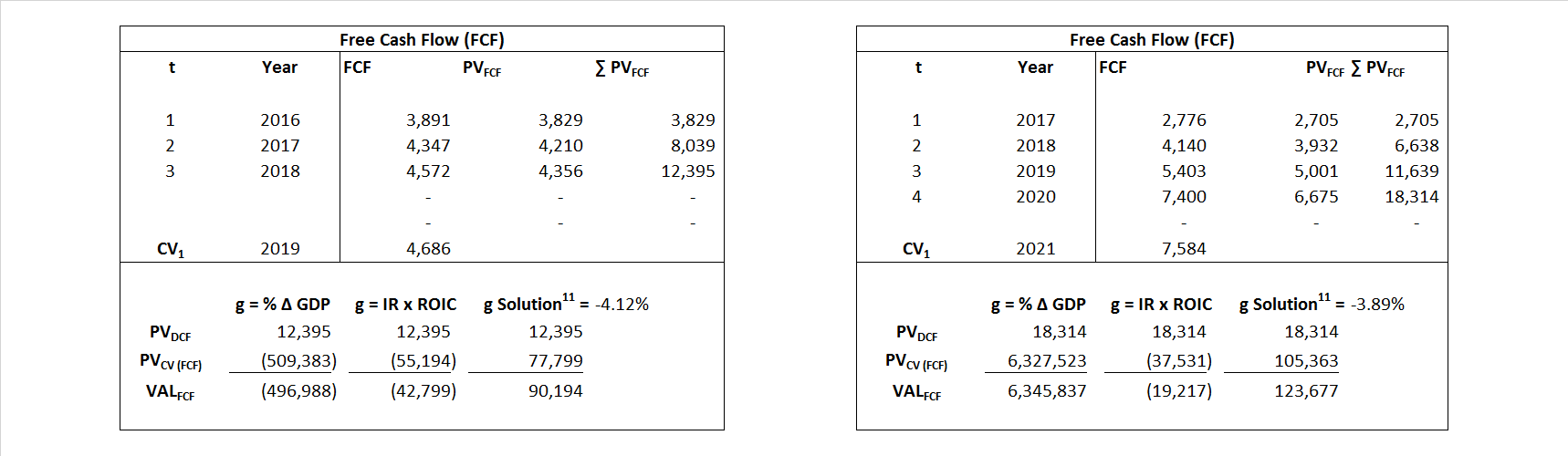Economic Profit ECON π${ Value }_{ { ECON\pi } }= I{ C }_{ 0 }+\sum { \frac { { IC }_{ t-1 }(ROI{ C }_{t}-WAC{C}_{t}) }{ { \left( 1+WACC \right) }^{ t } }+ \frac {\frac { I{C}_{0}\ x\ (ROI{C}_{1}\ -\ WAC{C}_{1}) }{ WACC-g } }{ { \left( 1+WACC \right) }^{ t } } }$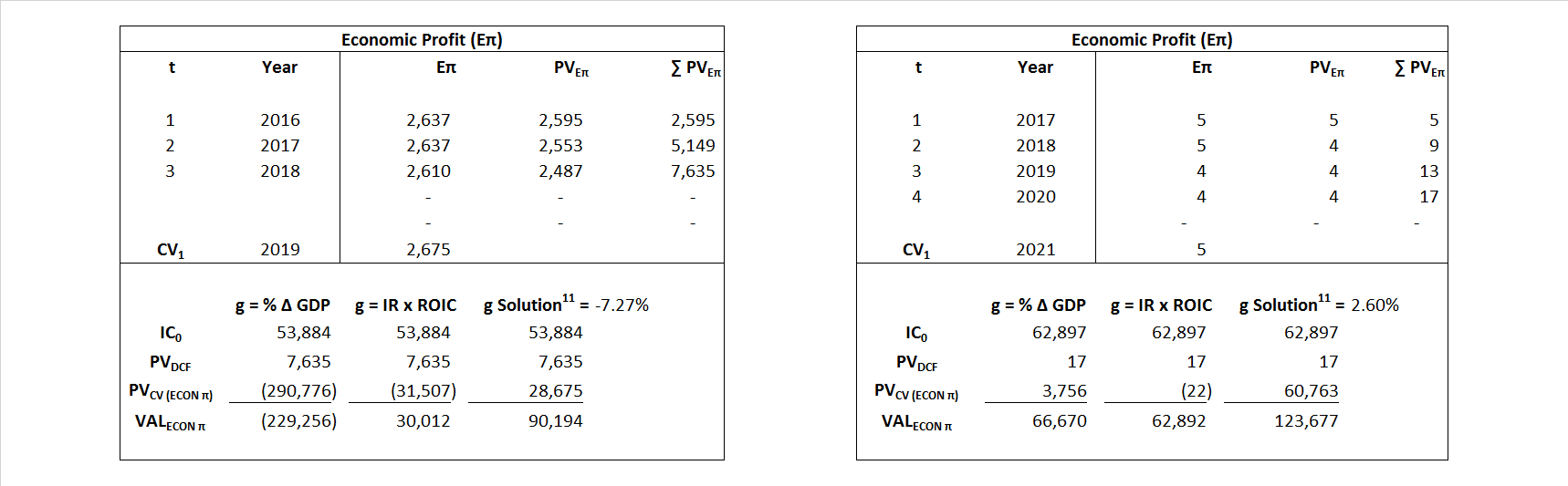Adjusted Present Value APV${ Value }_{ APV }=\sum { \frac { FCF_{ t } }{ { \left( 1+{ k }_{ u } \right) }^{ t } } +\frac { \frac { { FCF }_{ 1 }}{ { k }_{ u }-g } }{ { \left( 1+{ k }_{ u } \right) }^{ t } } } +\sum { \frac { { TS }_{ t } }{ { \left( 1+{ k }_{ tax } \right) }^{ t } } +\frac { \frac { { TS }_{ 1 }}{ { k }_{ tax }-g } }{ { \left( 1+{ k }_{ tax } \right) }^{ t } } }$Forward Market Multiple FMM${ Value }_{ DCF/FMM}=\sum { \frac { FCF_{ t } }{ { \left( 1+WACC \right) }^{ t } } +\frac { { EBIT }_{ 1 }\,{x}\,{FMM}}{ { \left( 1+WACC \right) }^{ t } } }{\,\,\,; \,\,FMM\,=\,\frac{{EV}_{t=0}}{{EBIT}_{t=0}}}$